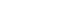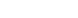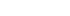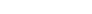## Chemistry Paper 2 Questions and Answers - Momaliche Post Mock 2020 Exam

INSTRUCTIONS TO CANDIDATES

• Answer all the questions in the spaces provided above.
• KNEC Mathematical tables and silent electronic calculators may be used.
• All working must be clearly shown where necessary.
• Candidates should answer the questions in English.
1.
1. Define the following terms:
1. Strong bases (1mk)
2. Amphoterism (1mk)
3. Solubility (1mk)
2. Using the equation below, identify the species that acts as the base in the forward reaction. Give a reason. (2mks)
NH3(g) + H2O(l)NH4+(aq) + OH-(aq)
3. A solution of ammonia gas in water causes a greater deflection of the ammeter whilesolution of ammonia gas in methylbenzene does not cause deflection. Explain this observation. (1mk)
4. Write a well-balanced chemical equation for the reaction between sodium hydroxide solution and zinc oxide. (1mk)
5. Explain how hard water is softened by ion exchange method. (2mks)
6. The table below gives the solubilities of sodium chloride and sodium sulphate at 0°C and 40°C.
 Substance Solubility in g/100g of water 0°C 40°C Sodium chloride 55 75 Sodium Sulphate 10 12

When an aqueous mixture containing 60g of sodium chloride and 7g of sodium sulphate in 100g of water at 80°C was cooled to 0°C, some crystals were observed.
1. Identify the crystals and determine the mass of the crystals formed. (2mks)
2. Name the method used to obtain the crystals. (1mk)
2.
1. Define
1. Molar heat of combustion. (1mk)
2. Heating value of a fuel. (1mk)
2. In an experiment to determine the heat of combustion of ethanol. CH3CH2OH, a student set up apparatus as shown in the diagram below. Study the set up and the data and answer the questions that follow.Volume of water = 100cm3
Final temperature of water = 36.0°c
Initial temperature of water = 22.0°c
Final mass of lamp an ethanol = 84.75g
Initial mass of lamp and ethanol = 85.10g
Density of water = 1g/cm3
(Specific heat capacity of water = 4.2kJKg-1K-1)
1. Calculate:
1. Number of moles of ethanol used in this experiment. (C=12, O=16, H=1)(1 mk)
2. The amount of heat given out in this experiment. (2mks)
3. The heat of combustion per mole of ethanol. (1 mk)
2. Write a thermochemical equation for the combustion of ethanol. (1 mk)
3. Explain how the molar heat of combustion for ethanol obtained above differs with the theoretical value. (2mks)
4. State one precaution that should be adhered to when carrying out this experiment. (1mk)
5. In this experiment an assumption that links ethanol and water is made. State the assumption.    (1 mk)
6. Draw an energy level diagram for the combustion of ethanol. (2mks)
3. The figure below represents a section of the periodic table. Study it and answer the questions that follow. Note that the letters do not represent the actual symbols of the elements.1. Consider elements H and I.
1. Explain why theatomic radius of element H is smaller than its ionic (1mk)
2. When element H was dissolved in water and blue litmus papers dipped, the colour of the litmus paper changed from blue to red to white. Explain. (2mks)
3. Explain what is likely to be observed when element H is bubbled through a solution containing the ions of element I. (2mks)
2. Compare the atomic radius of elements G and J. Explain the difference. (2mks)
3. Use dot and cross diagram to show bonding in a compound of B and H. (1mk)
4. G chloride has an unexpected bond type and structure.State the type of bond and the structure.
Bond type (lmk)
Structure (lmk)
5. A piece of blue litmus paper is placed in a solution of B chloride and a solution of G chloride. Explain what would be observed in each case.
1. B chloride solution (lmk)
2. G chloride solution (1mk)
4. In an experiment to study the rate of reaction, 2.5g of copper (II) sulphate crystals were added to a given mass of zinc granules and 100cm3 dilute hydrochloric acid at 27°C. The volume of hydrogen released was measured at 10 second intervals. The results obtained are tabulated below.
 Time (seconds) 0 10 20 30 40 50 60 70 80 90 Volume (cm3) 0 60 85 105 114 116 118 122 122 122

1. Why were the following not used in the reaction?
1. Nitric (V) acid (1mk)
2. Iron powder (1mk)
2. On the grid below plot a graph of volume of gas against time and label it X (3mks
1. Use the graph to calculate the rate of reaction at t=25seconds (2mks)
2. Explain why the volume of gas produced does not exceed 122cm3 (1mk)
3. Sketch graph Y on the same grid to show the results if the experiment is repeated at 20°C. (1mk)
4. How does the catalyst used (copper (II) sulphate) speed up the reaction? (1mk)
3.
1. State Le’Chatelier’s principle. (1mk)
2. What is the effect on the position of equilibrium when dilute hydrochloric acid is added to the closed system of the reaction below (1mk)
NH3 (g) + H2O (l)NH4+(aq) + OH-(aq)
5.
1. Give the IUPAC names of the following organic compounds. (2 mks)
1. CH3 – CH2 – CH – CH3
|
CH – CH3
|
CH3
2. CH3 – C ≡ C – CH3
2. Study the flow chart below and answer the questions that follow:1. Identify substances (2mks)
1. A
2. B
3. C
4. D
2. State how substance A and propanecould be distinguished chemically. (1 mk)
3. Give the components of soda lime in step I (2mks)
4. What is the industrial application of the process that converts substance A to propane?    (1mk)
5. In the laboratory propene can be prepared using propanol, broken porcelain and sodium hydroxide.
State the use of broken porcelain and sodium hydroxide solution
1. Broken porcelain (1mk)
2. Sodium hydroxide solution (1mk)
6.
1. Draw a fully labeled diagram of the apparatus you would use to electrolyse an electrolyte in the aqueous state. (3mks)
2. Explain why crystals of sodium chloride are non-conductorsof electricity butwhen melted they conducts electric current more readily. (2mk
3. Answer the following questions in relation to the electrolysis of molten lead (II) iodide.
1. State what happens to molten lead (II) iodide when an electric current is passed through it. (1mk)
2. At what electrode is a metal formed? Write an equation to show how the metal is formed. (2mks)
3. Why is it necessary to carry out this experiment in a fume chamber? (1mk)
4. What is a binary electrolyte? (1mk
4. Give the application of electrolysis in
1. Chemical manufacturing industry (1mk)
2. Metal extraction industries (1mk)
3. Jewellery e.g. necklaces manufacturing industries (1mk)
7. The diagram below represents the industrial manufacture of hydrochloric acid. Study it and answer the questions that follow.1. Name the main source of hydrogen in this process. (1mk)
2. The reaction between chlorine and hydrogen can be very explosive. How can this be avoided?   (1mk)
3. What is the role of glass beads in the absorption chamber? (1mk
4. Explain why the storage chamber for hydrochloric acid is made up of steel lined with rubber.    (1mk)
5. The acid obtained is 35% pure. Calculate its molarity, given that at 25°C, the density of the acid is 1.08g/cm3 (H=1, Cl=35.5) (3mks)
6. Explain why hydrochloric acid is not used to acidify potassium manganate (VII) solution.(1mk)## MARKING SHEME

1.
1. Define the following terms:
1. Strong bases (1mk)
• Bases that dissociate completely in water to yield more hydroxide ions
2. Amphoteric oxides (1mk)
• Oxides that react both as acids and bases
3. Solubility (1mk)
• The maximum mass of a solute required to saturate 100 g of the solvent at a particular temperature
2. Using the equation below, identify the species that acts as the base in the forward reaction. Give a reason. (2mks)
NH3(g) + H2O(l)NH4+(aq) + OH-(aq)
NH3; accepts a proton from water to form ammonium ion.
3. A solution of ammonia gas in water causes a greater deflection of the ammeter than a solution of ammonia gas in methylbenzene. Explain this observation. (1mk)
• In water ammonia dissolves and ionizes to produce hydroxide ions. In methylbenzene, ammonia dissolves but does not ionize to produce hydroxide ions.
4. Write a well-balanced chemical equation for the reaction between sodium hydroxide solution and zinc oxide. (1mk)
Zn(s)+ NaOH(aq) → Na2ZnO2(aq)+ H2O(l)
5. Explain how hard water is softened by ion exchange method. (2mks)
• The Ca2+ and Mg2+ ions in hard water are exchanged with Na+ in the column and precipitated out
6. The table below gives the solubilities of sodium chloride and sodium sulphate at 0°C and 40°C.
When an aqueous mixture containing 60g of sodium chloride and 7g of sodium sulphate in 100g of water at 80°C was cooled to 0°C, some crystals were observed.
1. Identify the crystals and determine the mass of the crystals formed. (2mks)
• Sodium chloride
Mass 60-55=5 g
2. Name the method used to obtain the crystals. (1mk)
• Fractional crystallization
2.
1. Define
1. Molar heat of combustion. (1mk)
• The enthalpy change/heat change when one mole of a substance is completely burned in oxygen
2. Heating value of a fuel. (1mk)
• The amount of heat energy given out when a unit mass or a unit volume of a fuel is completely burned in oxygen.
2. In an experiment to determine the heat of combustion of ethanol. CH3 CH2OH, a student set up apparatus as shown in the diagram below. Study the set up and the data and answer the questions that follow.
Volume of water = 100cm3
Final temperature of water = 36.0°c
Initial temperature of water = 22.00=°c
Final mass of lamp an ethanol = 84.75g
Initial mass of lamp and ethanol = 85.10g
Density of water = 1g/cm3
(Specific heat capacity of water = 4.2kJKg-1K-1)
1. Calculate:
1. Number of moles of ethanol used in this experiment. (C=12, O=16, H=1) (1 mk)
Mass of methanol = ( 85.10- 84.78) = 0.32 g
Moles = 0.32=0.01 moles
32
2. The amount of heat given out in this experiment. (2mks)
100×4.2 ×14 = 5880 J OR 5.88 k J
3. The heat of combustion per mole of ethanol. (1 mk)
0.01= 5.88
1= ?
1×5.88 = -588 KJ/mol
0.01
2. Write a thermochemical equation for the combustion of ethanol. (1 mk)
C2H5OH(l) + 3O2(g) → 2CO2(g) + 3H2O(g)   H=-588Kj/mol
3. Explain how the molar heat of combustion for ethanol obtained above differs with the theoretical value. (2mks)
• The molar heat of combustion is lower than the theoretical value due to heat lost to the surrounding or heat absorption by the apparatus
4. State one precaution that should be adhered to when carrying out this experiment. (1mk)
• The thermometer should not come into contact with the bottom of the hot beaker
5. In this experiment an assumption that links ethanol and water is made. State the assumption.  (1 mk)
• Heat released by the ethanol=heat gained by the water
6. Draw an energy level diagram for the combustion of ethanol. (2mks)3. The figure below represents a section of the periodic table. Study it and answer the questions that follow. Note that the letters do not represent the actual symbols of the elements.
1. Consider elements H and I.
1. Explain why the atomic radius of element H is smaller than its ionic radius (1mk)
• Due to electron-electron repulsion between the existing electrons and the incoming electron
2. When element H was dissolved in water and blue litmus papers dipped, the colour of the litmus paper changed from blue to red to white. Explain. (2mks)
• Dissolving element H in water form chlorine water which is acidic; the chloric (I) acid in chlorine water bleaches the litmus paper//Accept: A solution with bleaching properties
3. Explain what is likely to be the observation when element H is bubbled through a solution of element I. (2mks)
• The solution turns orange because element H displaces the ions of element I
2. Compare the atomic radius of of Element G and J. Explain the difference. (2mks)
• J has a smaller atomic radius than G; because it has more protons and hence a stronger nuclear force of attraction that pulls the energy levels closer to the nucleus.
3. Use dot and cross diagram to show bonding in a compound of B and H. (1mk)
• It is the ionic bond of NaCl
4. G chloride has an unexpected bond type and structure. State the type of bond and the structure.
• Bond type - Covalent/dative (lmk)
• Structure - Molecular (lmk)
5. A piece of blue litmus paper is placed in a solution of B chloride and a solution of G chloride. Explain what would be observed in each case.
1. B chloride solution (lmk)
• Remains blue; a solution of B chloride in water is neurtal
2. G chloride solution (1mk)
• Turns red; G chloride is hydrolysed in water to form an acidic solution
4. In an experiment to study the rate of reaction, 2.5g of copper (II) sulphate crystals were added to a given mass of zinc granules and 100 cm3 dilute hydrochloric acid at 25°C. The volume of hydrogen released was measured at 10 second intervals. The results obtained are tabulated below.
1. Why were the following not used in the reaction?
1. Nitric (V) acid (1mk)
• Oxidises hydrogen produced to water
2. Iron powder (1mk)
• Produces poisonous hydrogen sulphide gas
2. On the grid below plot a graph of volume of gas against time and label it X (3mks)1. Use the graph to calculate the rate of reaction at t=25seconds (2mks)
• A tangent must be done on the curve & its gradient determined
112-80  =0.275cm3/sec
130-14
2. Explain why the volume of gas produced does not exceed 122cm3 (1mk)
• As the reaction proceeds, hydrochloric acid and is being used up. When the volume is 122, all the acid has been used up.
3. Sketch graph Y on the same grid to show the results if the experiment is repeated at 10°C. (1mk)
4. How does the catalyst used (copper (II) sulphate) speed up the reaction? (1mk)
• By lowering activation energy; or by forming a short-lived intermediate product that decomposes to release the final products; or by providing a large surface area for effective collision of reacting particles. (accept 1 only).
3.
1. State Le’Chatelier’s principle. (1mk)
• When a change in condition is applied to a system in equilibrium, the system moves so as to oppose the change.
2. What is the effect on the position of equilibrium when dilute hydrochloric acid is added to the closed system of the reaction below (1mk)
NH3(g) + H2O(l)NH4+(aq) + OH-(aq)
• The position of equilibrium shifts to the right.
5.
1. Give the IUPAC names of the following organic compounds. (2 mks)
1. CH3 – CH2 – CH – CH3
|
CH – CH3
|
CH3
2,3-dimethylpentane
2. CH3 – C ≡ C – CH3
But-2-yne
2. Study the flow chart below and answer the questions that follow:
1. Identify substances (2 mks)
• A Propene
• B Sodium carbonate
• C Sodium butanoate
• D Propyl hydrogen sulphate
2. State how substance A and propanecould be distinguished chemically. (1 mk)
• A decolourises bromine water; propane does not. (accept use of dichromate or manganate)
3. Give the components of soda lime in step I (2mks)
• Sodium hydroxide and calcium oxide
4. What is the industrial application of the process that converts substance A to propane? (1mk)
• Manufacture of margarine/Hardening oils to fats
3. In the laboratory preparation of propene using propanol, broken porcelain and sodium hydroxide solution are used. State the use of broken porcelain and sodium hydroxide solution
1. Broken porcelain (1mk)
2. Sodium hydroxide solution (1mk)
• To remove carbon (VI) oxide and sulphur (VI) oxide formed when conc. sulphuric acid and ethanol decompose respectively due to heat.
6.
1. Draw a fully labeled diagram of the apparatus you would use to electrolyse an electrolyte in the aqueous state. (3mks)2. Explain why crystals of sodium chloride are non-conductors electricity but when melted they conducts electric current more readily. (2mk)
• Solid- Has fixed ions,
• Melt—Has mobile ions
3. Answer the following questions in relation to the electrolysis of molten lead (II) iodide.
1. State what happens to molten lead (II) iodide when an electric current is passed through it.
• It decomposes; Pb2+ migrate to the cathode while I- migrate to the anode (1mk)
2. At what electrode is a metal formed? Write an equation to show how the metal is formed. (2mks)
• Cathode
Pb2+(l) + 2e → Pb(s)
3. Why is it necessary to carry out this experiment in a fume chamber? (1mk)
• Iodine is poisonous
4. What is a binary electrolyte? (1mk)
• Contains only one type of cation and one type of anion
4. Give the application of electrolysis in
1. Chemical manufacturing industry (1mk)
• Manufacture of hydrogen, chlorine and sodium hydroxide
2. Metal extraction industries (1mk)
• Extract very reactive metals like Na & Al; Purify impure metals
3. Jewellery e.g. necklaces manufacturing industries (1mk)
• To electroplate to add beauty/ improve appearance
7. The diagram below represents the industrial manufacture of hydrochloric acid. Study it and answer the questions that follow.
1. Name the main source of hydrogen in this process. (1mk)
• Cracking of long chain alkanes or from natural gas
2. The reaction between chlorine and hydrogen can be very explosive. How can this be avoided?
• Pass hydrogen through a jet and burn in excess chlorine (1mk)
3. What is the role of glass beads in the absorption chamber? (1mk)
• Increase the surface area for the absorption of hydrogen chloride gas in water
4. Explain why the storage chamber for hydrochloric acid is made up of steel lined with rubber.
• Acid reacts with steel but not with rubber (1mk)
5. The acid obtained is 35% pure. Calculate its molarity, given that at 25°C, the density of the acid is 1.08g/cm3 (H=1, Cl=35.5) (3mks)
Concentration in g/litre
1 cmcontains 1.08g
1000=1000 × 1.08=1080g/litre
1
Molarity= 1080  = 29.59M
36.5
Molarity of pure acid = 35/100 × 29.59=10.36M
6. Explain why hydrochloric acid is not used to acidify potassium manganate (VII) solution.(1mk)
• It will be oxidized to chlorine by potassium manganate (VII).

• ✔ To read offline at any time.
• ✔ To Print at your convenience
• ✔ Share Easily with Friends / Students